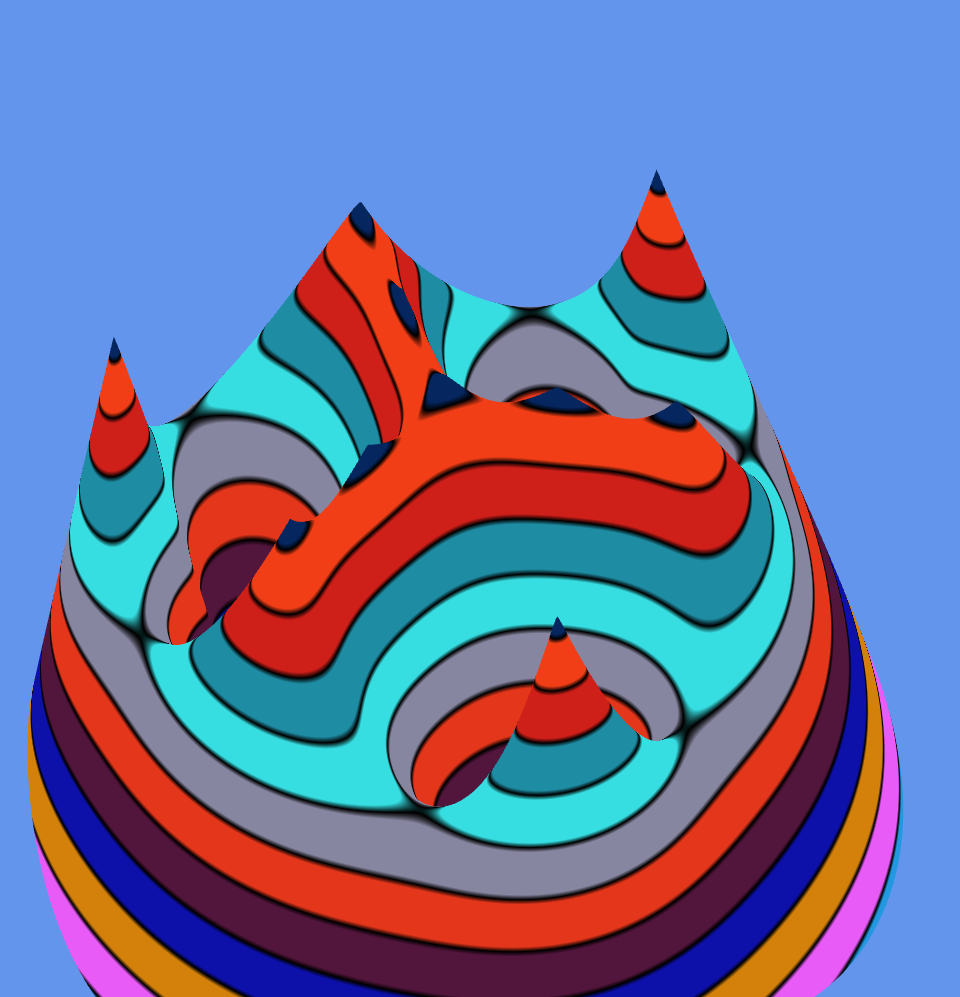## Thursday, October 2, 2014

### federgraph-special-case-number-one

In this blog post, I describe a problem or task. I do not give a solution, but I have already computed values in a simulation, so that you can look at the graphical output, before you start up your computer algebra software to create a new worksheet for the task.

It is about a system of springs. The Federgraph application has already implemented a 3D model of this system. The description of the task should be compatible with the identifiers used in the application, so that the mathematical model can stay in sync with the source code for the graph.

### The common case

A common case of a system of springs is given in the following picture. Three springs are attached to the corners of a triangle ABC. I have drawn circles to show the zero-force-length of each spring. The free end of each spring is connected to point P which can freely move within the (x, y) plane (where z = 0). We will consider the absolute value of force F, which keeps point P at a given location. We will also consider the amount of energy E stored within the system, when moved to point P.

﻿Picture 1: A system of springs

There are special locations, where force is zero and energy has a maximum or minimum. These locations are the stable or unstable equilibria, which we want to find as solutions of the equations of the mathematical model for the system. I can help out with some graphics.

### Special Case 1

This case is given when the triangle is equilateral, and the zero-length of each spring is equal to the height of the triangle.

As a special situation for special case 1, P shall be located at the midpoint of side AB, as depicted in the following picture.

﻿Picture 2: Schema view of special case 1

It is clear that the forces in two of the springs (AP, BP) cancel each other out, while the force in the third spring (CP) is zero, because the actual length of this spring (h) is equal to its zero-length (h). There happens to be an unstable equilibrium at point P. Think about it, then find A, B, C, and P in the following picture. This picture is a perspective projection, viewed directly from the top.

﻿﻿﻿
﻿Picture 3: Top view for special case 1

Question 1: How many equilibria exist? Perhaps it is easier to see in the next picture, which shows the same situation from a different angle.

﻿﻿
﻿Picture 4: Side view for special case 1

### Special Case 2

Now change the length of the springs, but keep them all at the same length (Parameter L). There is a bifurcation. It is easy to see when I scroll with the mouse wheel, but you should look at the math first. From the equations, you will know what to expect. It is a long time ago that I attended math lessons, I guess you are much better equipped than I am to analyze this stuff.
﻿﻿﻿
﻿Picture 5: Parameter L is close to where a bifurcation occurs
Question 2: At what value of parameter L does the bifurcation happen?

I hope that the term bifurcation is correct, not sure, but I leave the explanation of all this to you anyway.

### Special Case 3

The center point of the triangle is a special point. An equilibrium can be found there. We still work with the equilateral triangle, but in the following picture, the value of L is different again.
﻿Picture 6: Four solutions combined into one at the center point.﻿
Question 3: For what value of parameter L will the equilibrium at the center merge with three other special points?

### Conclusion

It is up to you to make conclusions. I have explored the system of springs, the general model, to some extent and do promise that there are more special cases. It will be fun to see them, once you have set up your worksheet!

This is my first blog post, I still have to find out how to do it properly. Perhaps I should not paste the text from Word, but instead paste clean Html? I will post a link to this blog-entry on my Profile and the Federgraph Page, where comments are enabled.